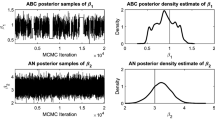# Algorithms to Find Exact Inclusion Probabilities for Conditional Poisson Sampling and Pareto πps Sampling Designs

## Abstract

Conditional Poisson Sampling Design as developed by Haje´k may be defined as a Poisson sampling conditioned by the requirement that the sample has fixed size. In this paper, an algorithm is implemented to calculate the conditional inclusion probabilities given the inclusion probabilities under Poisson Sampling. A simple algorithm is also given for second order inclusion probabilities in Conditional Poisson Sampling. Furthermore a numerical method is introduced to compute the unconditional inclusion probabilities when the conditional inclusion probabilities are predetermined. Simultaneously, we study the Pareto πps sampling design. This method, introduced by Rose´n, belongs to a class of sampling schemes called Order Sampling with Fixed Distribution Shape. Methods are provided to compute the first and second order inclusion probabilities numerically also in this case, as well as two procedures to adjust the parameters to get predetermined inclusion probabilities.

This is a preview of subscription content, log in via an institution to check access.

Price excludes VAT (USA)
Tax calculation will be finalised during checkout.

### Similar content being viewed by others### Approximate Bayesian computation using asymptotically normal point estimates

George Karabatsos### A simple introduction to Markov Chain Monte–Carlo sampling

Don van Ravenzwaaij, Pete Cassey & Scott D. BrownCheng-Hsien Li

## References

• N. Aires, “Exact Inclusion Probabilities for Conditional Poisson Sampling and Pareto pps Sampling Designs, ” Studies in Applied Probability and Statistics, Mathematical Statistics, Chalmers University of Technology, Göteborg University, 1998:5.

• N. Aires, “Comparisons between Conditional Poisson Sampling and Pareto pps Sampling Designs, ” Studies in Applied Probability and Statistics, Mathematical Statistics, Chalmers University of Technology, Göteborg University, (1999). Submitted to the Journal of Statistical Planning and Inference.

• K. Atkinson, An Introduction to Numerical Analysis, 2nd. ed., Wiley: Singapore, 1988.

• J. Dupačová, “A note on Rejective Sampling, ” Contributions to Statistics, Jaroslav Hájek Memorial Volume, Reidel: Holland and Academia: Prague, 71–78, 1979.

• J. Hájek, “Asymptotic Theory of Rejective Sampling with Varying Probabilities from a Finite Population, ” Annals of Mathematical Statistics vol. 35 pp. 1491–1523, 1964.

• J. Hájek, Sampling from a Finite Population, Marcel Dekker: New York, 1981.

• E. Ohlsson, “Sequential Poisson Sampling, ” Institute of Actuarial Mathematics and Mathematical Statistics, Stockholm University, Report No. 182, 1995.

• B. Rosén, “Asymptotic Theory for Order Sampling, ” Journal of Statistical Planning and Inference vol. 62 pp. 135–158, 1997.

• B. Rosén, “On Sampling with Probability Proportional to Size, ” Journal of Statistical Planning and Inference vol. 62 pp 159–191, 1997.

• B. Rosén, “On Inclusion Probabilities of Order Sampling, ” R&D Report, Research—Methods—Development 1998:2, Statistics Sweden, 1998.

• C. E. Särndal, B. Swensson, and J. Wretman, Model Assisted Survey Sampling, Springer Verlag: New York, 1992.

Authors

## Rights and permissions

Reprints and Permissions